# find general solution to dy/dx = e^-y(x - 2), what interval is this solution valid over?

find general solution to
dy/dx = e^-y(x - 2), what interval is this solution valid over?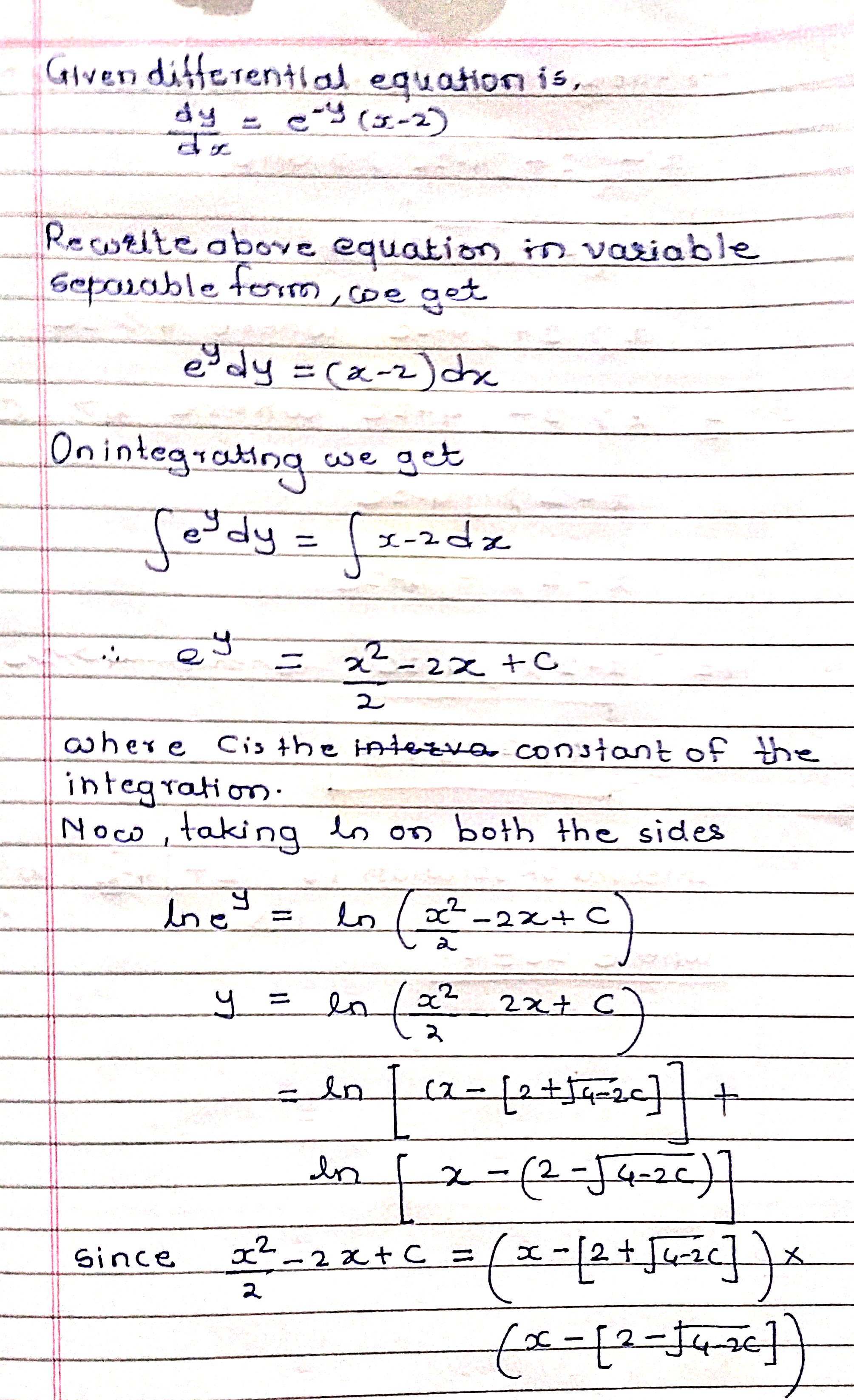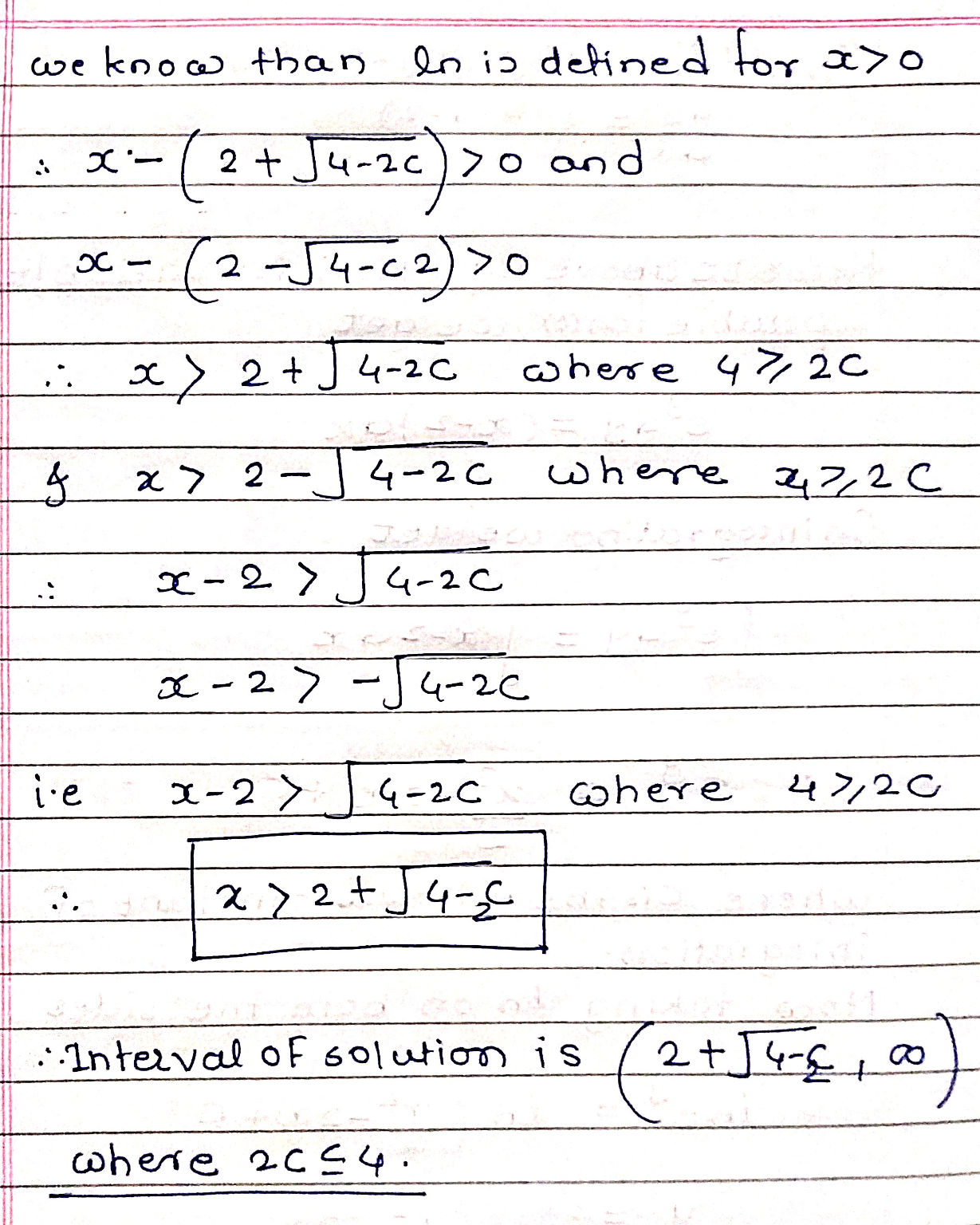##### Add Answer of: find general solution to dy/dx = e^-y(x - 2), what interval is this solution valid over?
Similar Homework Help Questions
• ### find general solution in implicit form of differential equation dy/dx= 2(e^x-e^-x)/y^2(e^x+e^-x)^4 (y>0)

find general solution in implicit form of differential equation dy/dx= 2(e^x-e^-x)/y^2(e^x+e^-x)^4 (y>0)

• ### (24 points) Find the general solution to each of the following differential equations dy a) =...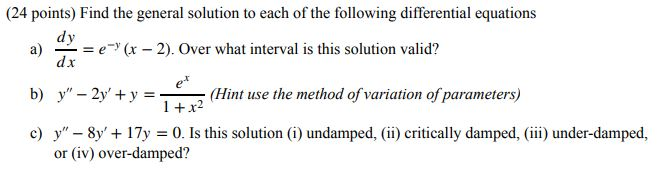(24 points) Find the general solution to each of the following differential equations dy a) = e)(x - 2). Over what interval is this solution valid? dx b) y" - 2y + y = (Hint use the method of variation of parameters) 1 + x2 c) y" - 8y' + 17y = 0. Is this solution (i) undamped, (ii) critically damped, (iii) under-damped, or (iv) over-damped?

• ### Find the general solution of dy/dx = (y/(x+?(xy))

Find the general solution of dy/dx = (y/(x+?(xy))

• ### 4. (24 points) Find the general solution to each of the following differential equations dy a)...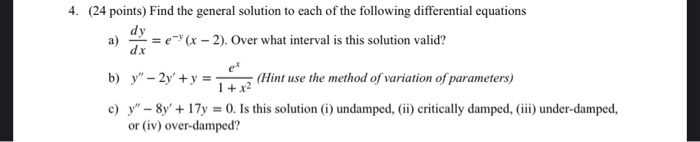4. (24 points) Find the general solution to each of the following differential equations dy a) = e-(x - 2). Over what interval is this solution valid? dx b) y" - 2y + y = (Hint use the method of variation of parameters) 1 + x2 c) y" - 8y' + 177 = 0. Is this solution (i)undamped, (ii) critically damped, (iii) under-damped, or (iv) over-damped?

• ### find general solution of (3x^2 + y + 3x^3y)dx + x dy = 0

find general solution of (3x^2 + y + 3x^3y)dx + x dy = 0 using exactness method

• ### Find the general solution of the following: x dy/dx +3y =x^3 -x y=?

Find the general solution of the following: x dy/dx +3y =x^3 -x y=?

• ### 210_8Q4 Consider the differential equation dy/dx=y/x^2' x > 0 Find the general solution of the differential...210_8Q4 Consider the differential equation dy/dx=y/x^2' x > 0 Find the general solution of the differential equation explicitly in the form y = f(x). Find the particular solution that satisfies y(1) = 1.

• ### Find dy/dx if y = (x^3)(e^2x)Find dy/dx if y = ln(x^2 + 5x)

Find dy/dx if y = (x^3)(e^2x)Find dy/dx if y = ln(x^2 + 5x)

• ### Find the general solution of the given differential equation. dy 324y 8 dx ytx) Give the largest interval I over which the general solution is defined. (Think about the implications of any singul...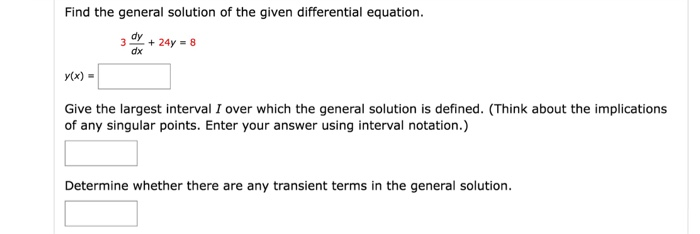Find the general solution of the given differential equation. dy 324y 8 dx ytx) Give the largest interval I over which the general solution is defined. (Think about the implications of any singular points. Enter your answer using interval notation.) Determine whether there are any transient terms in the general solution. Find the general solution of the given differential equation. dy 324y 8 dx ytx) Give the largest interval I over which the general solution is defined. (Think about the...

• ### it y=x+ 1/ / is the general solution of dy dx (y – x)2 +1, then...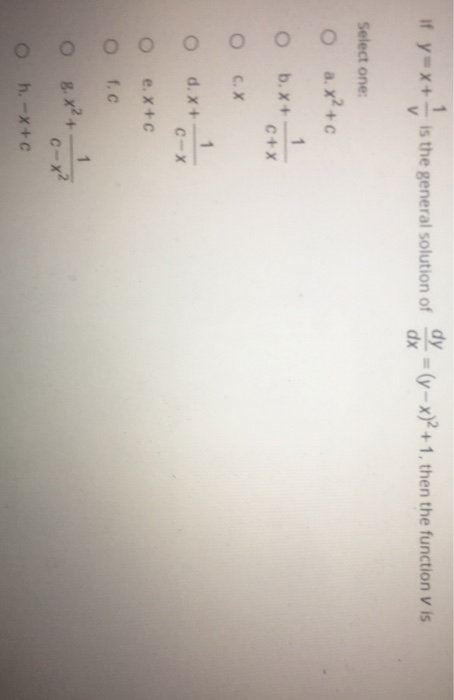it y=x+ 1/ / is the general solution of dy dx (y – x)2 +1, then the function v is Select one O a.x²+c 1 o b. x+ C+X cox o d. x + CX e. X + f.c 1 0 8.x²+ c-x? O h-x+c

Free Homework App